# LAKHMIR SINGH AND MANJIT KAUR Solutions for Class 9 Physics Chapter 3 - Gravitation

Page / Exercise

## Chapter 3 - Gravitation Exercise 100

Question 1

What is the value of gravitational constant G (i) on the earth, and (ii) on the moon?

Solution 1

Value of gravitational constant G on the earth and the moon is = 6.67 x 10-11Nm2/kg2

Note that the value of G always remains constant irrespective of the location.

Question 2

Which force is responsible for the moon revolving round the earth?

Solution 2

Gravitational force is responsible for the moon revolving round the earth.

Question 3

Does the acceleration produced in a freely falling body depend on the mass of the body?

Solution 3

No, the acceleration produced in a freely falling body is independent of the mass of the body.

Question 4

Name the scientist who gave the three laws of planetary motion.

Solution 4

Johannes Kepler gave the three laws of planetary motion.

Question 5

Name the scientist who explained the motion of planets on the basis of gravitational force between the sun and planets.

Solution 5

Newton explained the motion of planets on the basis of gravitational force between the sun and planets.

Question 6

State the Kepler's Law which is represented by the relation.

Solution 6

Kepler's law of periods states that: The cube of the mean distance of a planet from the sun is directly proportional to the square of time it takes to move around the sun.

Question 7

Which of the Kepler's law of planetary motion led Newton to establish the inverse-square rule for gravitational force between two bodies?

Solution 7

Kepler's third law of planetary motion led Newton to establish the inverse-square rule for gravitational force between two bodies.

Question 8

Name the property of earth which is responsible for extremely small acceleration being produced in it as a result of attraction by other small objects..

Solution 8

Extremely large mass of the earth.

Question 9

What is the acceleration produced in a freely falling body of mass 10kg? (Neglect air resistance)

Solution 9

Acceleration produced in a freely falling body, irrespective of its mass, is 9.8m/s2

Question 10

When an object is dropped from a height, it accelerates and falls down. Name the force which accelerates the object.

Solution 10

Gravitational force of the earth.

Question 11

Give the formula for the gravitational force F between two bodies of masses M and m kept at a distance d from each other.

Solution 11

The gravitational force F between two bodies of masses M and m kept at a distance d from each other is :Here, Gravitational constant, G=6.7 x10-11Nm2 kg-2

Question 12

What force is responsible for the earth revolving round the sun?

Solution 12

Gravitational force is responsible for the earth revolving round the sun.

Question 13

What name has been given to the force with which two objects lying apart attract each other?

Solution 13

Gravitational force causes two objects lying apart attract each other.

Question 14

What type of force is involved in the formation of tides in the sea?

Solution 14

Gravitational force (exerted mainly by the moon and to some extent by the sun) is involved in the formation of tides in the sea.

Question 15

Which force is responsible for holding the solar system together?

Solution 15

Gravitational force of the sun holds the solar system together.

Question 16

What is the weight of a 1kilogram mass on the earth? (g=9.8m/s2)

Solution 16

Weight, W = m x g

= 1 kg x 9.8m/s2=9.8 N

Question 17

On what factor/factors does the weight of a body depend?

Solution 17

The weight of a body is directly proportional to its mass.

It also depends on the acceleration due to gravity which varies from place to place.

Question 18

As the altitude of a body increases, do the weight and mass both vary?

Solution 18

Weight of the body varies with altitude; mass of an object is constant.

Question 19

If the same body is taken to places having different gravitational field strength, then what will vary: its weight or mass?

Solution 19

Its weight varies; mass of an object is constant.

Question 20

If the mass of an object be 10kg, what is its weight? (g=9.8m/s2)

Solution 20

Weight, W=m x g

= 10 x 9.8 =98 N

Question 21

The weight of a body is 50N. What is its mass? (g=9.8m/s2)

Solution 21

Weight, W=m x gQuestion 22

A body has a weight of 10kg on the surface of earth. What will be its weight when taken to the centre of the earth?

Solution 22

Its weight will be zero as value of g is zero at the centre of the earth.

Question 23

Write down the weight of a 50 kg mass on the earth. (g=9.8m/s2)

Solution 23

Weight, W=m x g

=50 x 9.8=490N

Question 24

If the weight of a body on the earth is 6N, what will it be on the moon?

Solution 24

Weight of the body on the surface of moon will be 1N approx. as the value of g on the surface of moon is one-sixth that of the earth.

Question 25

State whether the following statements are true or false:

(a) A falling stone also attracts the earth.

(b) The force of gravitation between two objects depends on the nature of medium between them.

(c) The value of G on the moon is about one-sixth of the value of G on the earth.

(d) The acceleration due to gravity acting on a freely falling body is directly proportional to the mass of the body.

(e) The weight of an object on the earth is about one-sixth of the weight on the moon.

Solution 25

(a) True

(b) False

(c) False

(d) False

(e) False

## Chapter 3 - Gravitation Exercise 101

Question 26

Fill in the blanks with suitable words:

(a) The acceleration due to gravity on the moon is about______ of that on the earth.

(b) In order that the force of gravitation between two bodies may become noticeable and cause motion, one of the bodies must have an extremely large _______.

(c) The weight of an object on the earth is about _____of its weight on the moon.

(d) The weight of an object on the moon is about ______of its weight on the earth.

(e) The value of g on the earth is about _____ of that on the moon.

(f) If the weight of a body is 6N on the moon, it will be about _____ on the earth.

Solution 26

(a) One-sixth

(b) Mass

(c) Six times

(d) One-sixth

(e) Six times

(f) 36N

Question 27

Explain what is meant by the equation:Where the symbols have their usual meanings.

Solution 27

This is the acceleration produced by the earth. It is also called acceleration due to gravity.where, G= gravitational constant

M= mass of the earth.

Question 28

(a)What do you mean by the term 'free fall'?

(b)During a free fall, will heavier objects accelerate more than the lighter ones?

Solution 28

(a)The falling of a body from a height towards the Earth under the gravitational force of the Earth (with no other forces acting on it) is called free fall.

(b)No, acceleration is independent of the mass of the body during free fall.

Question 29

Can we apply Newton's third law to the gravitational force? Explain your answer.

Solution 29

Yes, Newton's third law of motion holds good for the force of gravitation. This means that when earth exerts a force of attraction on an object, then the object also exerts an equal force on the earth, in the opposite direction.

Question 30

Give reason for the following:

The force of gravitation between two cricket balls is extremely small but that between a cricket ball and the earth is extremely large.

Solution 30

The force of gravitation between two bodies is directly proportional to the product of their masses.Since the mass of cricket balls is very small as compared to that of the earth, so the force of gravitation between two cricket balls is extremely small while that between a ball and the earth is extremely large.

Question 31

Describe how the gravitational force between two objects depends on the distance between them.

Solution 31

The gravitational force F between two bodies of masses M and m kept at a distance d from each other is :The force between two bodies is inversely proportional to the square of the distance between them. That is,Therefore , if we double the distance between two bodies, the gravitational force becomes one-fourth and if we halve the distance between two bodies, then the gravitational force becomes four times.

Question 32

What happens to the gravitational force between two objects when the distance between them is:

(i) Doubled?

(ii) Halved?

Solution 32

(a) If we double the distance between two bodies, the gravitational force becomes one-fourth.

(b) If we halve the distance between two bodies, then the gravitational force becomes four times.

Question 33

State two applications of universal law of gravitation.

Solution 33

(i) Universal law of gravitation is used to determine the masses of the sun, the earth and the moon accurately.

(ii) Universal law of gravitation helps in discovering new stars and planets.

Question 34

Explain why, if a stone held in our hand is released, it falls towards the earth.

Solution 34

This is because the earth exerts a force of attraction (called gravity) on the stone and pulls it down.

Question 35

Calculate the force of gravitation between two objects of masses 50kg and 120kg respectively kept at a distance of 10 m from one another.(gravitational constant, G=6.7 x 10-11 Nm2/kg2)

Solution 35m=50kg

M=120kg

Distance, d=10m

G=6.7 x 10-11 Nm2/kg2Question 36

What is the force of gravity on a body of mass 150 kg lying on the surface of the earth? (mass of earth = 6 x 1024kg; Radius of earth= 6.4 x 106m; G=6.7 x 10-11 Nm2/kg2)

Solution 36

Force due to gravity,Question 37

The mass of sun is 2 x1030kg and the mass of earth is 6 x 1024kg. If the average distance between the sun and the earth be 1.5 x 108km, calculate the force of gravitation between them.

Solution 37

Distance d=1.5 x 108 km= 1.5 x 1011 m

Mass of the sun, m=2 x1030 kg

Mass of the earth, M= 6 x 1024kg

Force of gravitation,Question 38

A piece of stone is thrown vertically upwards. It reaches the maximum height in 3 seconds. If the acceleration of the stone be 9.8 m/s2 directed towards the ground, calculate the initial velocity of the stone with which it is thrown upwards.

Solution 38

Initial velocity of the stone, u=?

Final velocity of stone, v=0

Acceleration due to gravity, g= -9.8m/s2

Time, t=3 sec

Using relation, v=u + gt

0 = u -9.8 x 3

u =29.4m/s

Question 39

A stone falls from a building and reaches the ground 2.5 seconds later. How high is the building? (g=9.8m/s2)

Solution 39

Initial velocity, u=0m/s

Acceleration due to gravity, g=9.8m/s2

Time taken to reach the ground, t=2.5 sec

Height, h=?

Using relation,Question 40

A stone is dropped from a height of 20 m.

(i) How long will it take to reach the ground?

(ii) What will be its speed when it hits the ground? (g=10m/s2)

Solution 40

Height, s=20m

Initial velocity, u=0

Acceleration due to gravity, g=10m/s2

Final velocity, v=?

Time taken, t=?

(i) Using relation,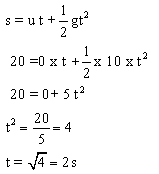(ii) For a freely falling body:

v2 = u2 + 2gh

= (0)2 + 2 x (10) x (20)

So, v2 =400The speed of stone when it hits the ground will be 20m/s.

Question 41

A stone is thrown vertically upwards with a speed of 20m/s. How high will it go before it begins to fall? (g=9.8m/s2)

Solution 41

Initial velocity, u=20m/s

Final velocity, v=0

Acceleration due to gravity, g=-9.8m/s2

Height, h=?

Using relation, for a freely falling body:

v2 = u2 + 2gh

(0)2 = (20)2 + 2 x (-9.8) x h

0-400 = -19.6 h

h= 400/19.6 = 20.4 m

Question 42

When a cricket ball is thrown vertically upwards, it reaches a maximum height of 5 metres.

(a) What was the initial speed of the ball?

(b) How much time is taken by the ball to reach the highest point? (g=10m/s2)

Solution 42

Initial velocity, u=?

Final velocity, v=0

Acceleration due to gravity, g=-10m/s2

Height, h=5 m

(a) For a freely falling body:

v2 = u2 + 2gh

(0)2 = u2+ 2 x(-10)x 5

0= u2 -100

u2= 100

So, u=10m/s

(b) Using relation, v=u + gt

0 = 10 + (-10) t

-10= -10 t

t=1sec

Question 43

Write the differences between mass and weight of an object.

Solution 43
 Mass Weight 1. The mass of an object is the quantity of matter contained in it. 1. The weight of an object is the force with which it is attracted towards the centre of the earth. 2. SI unit of mass is kilogram (kg). 2. SI unit of mass is newton (N). 3. The mass of an object is constant. 3. The weight of an object is not constant. It changes with the change in acceleration due to gravity. 4. The mass of an object can never be zero. 4. The weight of an object can be zero.

Question 44

Can a body have mass but no weight? Give reasons for your answer.

Solution 44

Yes, weight of a body is not constant, it varies with the value of acceleration due to gravity, g.

Weight of a body is zero, when it is taken to the centre of the earth or in the interplanetary space, where g=0.

## Chapter 3 - Gravitation Exercise 102

Question 45

A force of 20N acts upon a body whose weight is 9.8N. What is the mass of the body and how much is its acceleration? (g=9.8m/s2)

Solution 45

Weight= 9.8N

W= m x g

9.8 =m x 9.8

m= 1kg

Force, F= mass x acceleration

20 N = 1kg x a

Acceleration, a=20m/s2

Question 46

A stone resting on the ground has a gravitational force of 20 N acting on it. What is the weight of the stone? What is its mass? (g=10m/s2).

Solution 46
Weight of the stone = Gravitational force acting on it = 20 N

Weight, W= m x g

20=m x 10

m=2 kg

Question 47

An object has mass of 20kg on earth. What will be its (i) mass, and (ii) weight, on the moon? (g on moon=1.6 m/s2)

Solution 47

(i) Its mass will be 20 kg as mass is a constant quantity.

(ii) Weight, W= m x g

=20 x 1.6=32 N

Question 48

Which is more fundamental, the mass of a body or its weight? Why?

Solution 48

The mass of a body is more fundamental because mass of a body is constant and does not change from place to place.

Question 49

How much is the weight of an object on the moon as compared to its weight on the earth? Give reason for your answer.

Solution 49

The weight of an object on the moon is about one-sixth of its weight on the earth. This is because the value of acceleration due to gravity on the moon is about one-sixth of that on the earth.

Question 50

(a) Define mass of a body. What is the SI unit of mass?

(b) Define weight of a body. What is the SI unit of weight?

(c) What is the relation between mass and weight of a body?

Solution 50

(a) The mass of a body is the quantity of matter contained in it. The SI unit of mass is kilogram (kg).

(b) The weight of a body is the force with which it is attracted towards the centre of the earth. The SI unit of weight is newton (N).

(c) Weight, W =m x g, i.e. the weight of a body is directly proportional to its mass.

Question 51

(a) State the universal law of gravitation. Name the scientist who gave this law.

(b) Define gravitational constant. What are the units of gravitational constant?

Solution 51

(a) According to universal law of gravitation: Every body in the universe attracts every other body with a force (F) which is directly proportional to the product of their masses (m and M) and inversely proportional to the square of the distance (d) between them.Sir Isaac Newton gave this law.

(b) The gravitational constant G is numerically equal to the force of gravitation which exists between two bodies of unit masses kept at a unit distance from each other.Units of gravitational constant= Nm2/kg2

Question 52

(a) What do you understand by the term 'acceleration due to gravity of earth'?

(b) What is the usual value of acceleration due to gravity of earth?

(c) State the SI unit of acceleration due to gravity.

Solution 52

(a) The uniform acceleration produced in a freely falling body due to the gravitational force of the earth is called acceleration due to gravity of earth.

(b) Usual value of acceleration due to gravity, g=9.8 m/s2.

(c) SI unit of acceleration due to gravity is m/s2.

Question 53

(a) Is the acceleration due to gravity of earth 'g' a constant? Discuss.

(b) Calculate the acceleration due to gravity on the surface of a satellite having a mass of 7.4 x 1022kg and a radius of 1.74 x 106m (G= 6.7 x 10-11 Nm2/kg2). Which satellite do you think it could be?

Solution 53

(a) No, the value of acceleration due to gravity (g) is not constant at all the places on the surface of the earth. Since the radius of the earth is minimum at the poles and maximum at the equator, the value of g is maximum at the poles and minimum at the equator. As we go up from the surface of the earth, the distance from the centre of the earth increases and hence the value of g decreases. The value of g also decreases as we go down inside the earth.

(b) Acceleration due to gravity,Mass, M= 7.4 x 1022kg

Gravitational constant, G= 6.7 x 10-11Nm2/kg2As the value of g =1.637m/s2, which is one sixth the value of g on earth, the satellite could be moon.

Question 54

State and explain Kepler's laws of planetary motion. Draw diagrams to illustrate these laws.

Solution 54

Kepler's first law: The planets move in elliptical orbits around the sun, with the sun at one of the two foci of the elliptical orbit. This law means that the orbit of a planet around the sun is an ellipse and not an exact circle. An elliptical path has two foci, and the sun is at one of the two foci of the elliptical path.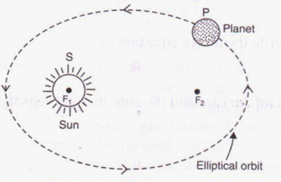Kepler's Second law states that: Each planet revolves around the sun in such a way that the line joining the planet to the sun sweeps over equal areas in equal intervals of time. This means that a planet does not move with constant speed around the sun. The speed is greater when the planet is nearer the sun, and less when the planet is farther away from the sun.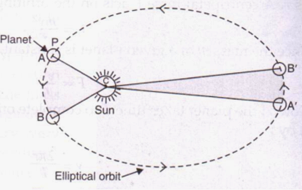Kepler's Third Law states that: The cube of the mean distance of a planet from the sun is directly proportional to the square of time it takes to move around the sun.Question 55

The mass of a planet is 6 x 1024 kg and its diameter is 12.8 x103 km. If the value of gravitational constant be 6.7 x 10-11 Nm2/kg2, calculate the value of acceleration due to gravity on the surface of the planet. What planet could this be?

Solution 55

Acceleration due to gravity,Mass, M = 6x 1024kg

Diameter = 12.8 x 103 km = 12.8 x 106 m

Radius, R = (12.8 x 106)/2 =6.4x 106m

Gravitational constant, G= 6.7 x 10-11Nm2/kg2As the value of g=9.8m/s2, the planet could be Earth.

## Chapter 3 - Gravitation Exercise 103

Question 71

If the distance between two masses is increased by a factor of 5, by what factor would the mass of one of them have to be altered to maintain the same gravitational force? Would this be an increase or decrease in the mass?

Solution 71

Gravitational force is given by:Distance between two masses is increased s.t. new distance is D= 5 d

New gravitational force F1 = F

Let on of the mass is changed to m1 so as to maintain the same gravitational force.m1 = 25m

Hence one of the masses should be increased by 25 times in order to have the same gravitational force.

Question 72

Universal law of gravitation states that every object exerts a gravitational force of attraction on every other object. If this is true, why don't we notice such forces? Why don't the two objects in a room move towards each other due to this force?

Solution 72

In order to be able to notice the gravitational force of attraction between any two objects, at least one of the objects on the earth should have an extremely large mass. Since no object on the earth have an extremely large mass, we cannot notice such forces.

The two objects in a room do not move towards each other because due to their small masses, the gravitational force of attraction between them is very, very weak.

Question 73

Suppose a planet exists whose mass and radius both are half those of the earth. Calculate the acceleration due to gravity on the surface of this planet.

Solution 73

Acceleration due to gravity of earth,If mass of planet, m= M/2

And radius of planet, r= R/2

Acceleration due to gravity on the surface of planet will be:## Chapter 3 - Gravitation Exercise 104

Question 74

A coin and a piece of paper are dropped simultaneously from the same height. Which of the two will touch the ground first? What will happen if the coin and the piece of paper are dropped in vacuum? Give reason for your answer.

Solution 74

The coin reaches the ground first as compared to the piece of paper because it experiences lesser resistance from air than that felt by paper.

If the coin and the piece of paper are dropped in vacuum, both of them will touch the ground at the same time.

Question 75

A stone and the earth attract each other with an equal and opposite force. Why then we see only the stone falling towards the earth but not the earth rising towards the stone?

Solution 75

The mass of a stone is very small, due to which the gravitational force produces a large acceleration in it. Due to large acceleration of stone, we see stone falling towards the earth. The mass of earth is, however, very, very large. Due to the very large mass of the earth, the same gravitational force produces very, very small acceleration in the earth, that it cannot be observed. And hence we do not see the earth rising up towards the stone.

Question 76

What is the actual shape of the orbit of a planet around the sun? What assumption was made by Newton regarding the shape of an orbit of a planet around the sun for deriving his inverse square rule from Kepler's third law of planetary motion?

Solution 76

The actual shape of the orbit of a planet around the sun is elliptical.

The assumption made by the Newton regarding the shape of an orbit of a planet around the sun was that the orbit of a planet around the sun is 'circular'.

Question 77

The values of g at six distances A, B, C, D, E and F from the surface of the earth are found to be 3.08m/s2,9.23m/s2 ,0.57 m/s2, 7.34 m/s2 , 0.30 m/s2 , and 1.49 m/s2 , respectively.

(a) Arrange these values of g according to the increasing distances from the surface of the earth (keeping the value of g nearest to the surface of the earth first)

(b) If the value of distance F be 10000km from the surface of the earth, state whether this distance is deep inside the earth or high up in the sky? Give reason for your answer.

Solution 77

(a) 9.23 m/s2 , 7.34 m/s2 , 3.08 m/s2 , 1.49 m/s2 , 0.57 m/s2 , 0.30 m/s2

(b) This distance F of 10000 km is high up in the sky. The distance of 10000 km cannot be deep inside the earth because the radius of earth is only about 6400km and the value of g at the centre of earth becomes zero.

## Chapter 3 - Gravitation Exercise 123

Question 1

Write the common unit of density.

Solution 1

Grams per cubic centimtre (g/cm3).

Question 2

What is the density of water in SI units?

Solution 2

Density of water =1000kg/m3.

Question 3

What is the value of relative density of water?

Solution 3

Relative density of water is 1.

Question 4

Name the quantity whose one of the units is Pascal (Pa).

Solution 4

Pressure has unit of Pascal (Pa).

Question 5
State the units in which pressure is measured.
Solution 5
Pressure is measured in newtons per square metre (N/m2) i.e., pascal (Pa).
Question 6

State whether the following statements are true or false:

(a) The buoyant force depends on the nature of object immersed in the liquid.

(b) Archimedes' principle can also be applied to gases.

Solution 6

(a) False

(b) True

Question 7

In which direction does the buoyant force on an object due to a liquid act?

Solution 7

Buoyant force on an object due to a liquid acts in the vertically upward direction.

Question 8

What is the other name of buoyant force?

Solution 8

Upthrust is the other name of buoyant force.

Question 9

Name the force which makes heavy objects appear light when immersed in a liquid.

Solution 9

Buoyant force.

Question 10

What is upthrust?

Solution 10

The upward force acting on an object immersed in a liquid is called upthrust.

Question 11

Name the principle which gives the magnitude of buoyant force acting on an object immersed in a liquid.

Solution 11

Archimedes' Principle.

Question 12

The relative density of mercury is 13.6. What does this statement mean?

Solution 12

The relative density of mercury is 13.6, this means that mercury is 13.6 times as heavy as an equal volume of water.

Question 13

What name is given to 'thrust per unit area'?

Solution 13

Pressure is 'thrust per unit area'.

Question 14

What is the scientific name of the 'upward force' acting on a object immersed in a liquid?

Solution 14

Buoyant force or upthrust.

Question 15

What is meant by the term 'Buoyancy'?

Solution 15

The tendency of a liquid to exert an upward force on an object placed in it, is called buoyancy.

Question 16

What causes buoyant force (or upthrust) on a boat?

Solution 16

The buoyant force on a boat is caused by the pressure of water 'pushing up' on the bottom of the boat.

Question 17

Why does ice float in water?

Solution 17

The density of ice is less than that of water, so ice floats in water.

Question 18

What force acting on an area of 0.5 m2 will produce a pressure of 500 Pa?

Solution 18Question 19

An object of weight 200N is floating in a liquid. What is the magnitude of buoyant force acting on it?

Solution 19

Since the object floats in the liquid, so the magnitude of the buoyant force exerted by the liquid is equal to the weight of the object.

Hence, buoyant force =200N

Question 20

Name the scientist who gave the magnitude of buoyant force acting on a solid object immersed in a liquid.

Solution 20

Archimedes gave the magnitude of buoyant force acting on a solid object immersed in a liquid

Question 21

The density of gold is 19g/cm3. Find the volume of 95 g of gold.

Solution 21Question 22

What is the mass of 5m3 of cement of density 3000kg/m3?

Solution 22

Volume=5m3

Density= 3000kg/m3Question 23

What is the density of a substance of mass 100g and volume 10 cm3?

Solution 23

Mass of the substance = 100g

Volume of the substance =10cm3Question 24

Why does a block of wood held under water rise to the surface when released?

Solution 24
Because the weight of the block of wood is less than the weight of an equal volume of water. So when it is completely submerged in water, the upward buoyant force on it is greater than the downward gravitational force on it. Hence, the lock rises to the surface.

Question 25

The density of a body is 800kg/m3. Will it sink or float when dipped in a bucket of water? (Density of water=1000kg/m3).

Solution 25

The body will float when dipped in a bucket of water as its density is less than that of water.

Question 26

Fill in the blanks:

(a) Force acting on a unit area is called_____.

(b) It is the _____ force which makes objects appear lighter in water.

(c) A heavy ship floats in water because its _____ density is less than that of water.

(d) In fluids (liquids or gases), pressure acts in ____ directions, and pressure ______as the depth increases.

(e) In order to sink in a fluid, the density of an object must be_____ than the ____ of the fluid.

(f) Snow shoes work by spreading out a person's ____ over a much bigger _______.

(g) If the area of a snow shoe is five times ____than the area of an ordinary shoe, then the pressure of a snow shoe on the snow is five times _____.

Solution 26

(a) pressure

(b) buoyant

(c) average

(d) all; increases

(e) less; density

(f) weight; area

(g) bigger; smaller

## Chapter 3 - Gravitation Exercise 124

Question 27

(a) What is the difference between the density and relative density of a substance?

(b) If the relative density of a substance is 7.1, what will be its density in SI units?

Solution 27

(a)          The density of a substance is defined as mass of the substance per unit volume.SI unit of density is kg/m3.

The relative density of a substance is the ratio of its density to that of water.It has no units.

(b)Question 28

Define thrust. What is its unit?

Solution 28

The force acting on a body perpendicular to its surface is called thrust.

The SI unit of thrust is newton (N).

Question 29

A mug full of water appears light as long as it is under water in the bucket than when it is outside water. Why?

Solution 29

A mug full of water appears light as long as it is under water because buoyant force acts on it which reduces its effective weight and makes it appear lighter.

Question 30

What happens to the buoyant force as more and more volume of a solid object is immersed in a liquid? When does the buoyant force become maximum?

Solution 30

As more and more volume of the solid object is immersed in the liquid, the upward 'buoyant force' also keeps on increasing. When the object is completely immersed in the liquid, the buoyant force acting on the solid becomes maximum and remains constant thereafter.

Question 31

Why do we feel light on our feet when standing in a swimming pool with water up to our armpits?

Solution 31

As more and more volume of our body is immersed in water, the apparent weight of the body goes on decreasing and the body seems to become lighter. This is due to the increase in upward buoyant force acting on the body.

Question 32

Explain why, big boulders can be moved easily by flood.

Solution 32

Big boulders weig much less while in water and as such are easily moved by the flood.

Question 33

An iron nail sinks in water but it floats in mercury. Why?

Solution 33

An iron nail sinks in water but it floats in mercury because density of iron is more than that of water but less than that of mercury.

Question 34

Explain why, a piece of glass sinks in water but it floats in mercury.

Solution 34

A piece of glass sinks in water but it floats in mercury because density of glass is more than that of water but less than that of mercury.

Question 35

Steel pin sinks in water but a steel boat floats. Why?

Solution 35

A piece of steel sinks in water because steel is denser than water. However, a steel ship is a hollow object made of steel and contains a lot of air in it. Due to presence of a lot of air in it, the average density of the ship becomes less than the density of water. Hence a ship floats in water.

Question 36

Explain why, school bags are provided with wide straps to carry them.

Solution 36

School bags have wide straps so that their weight may spread over a large area of shoulder producing less pressure on the shoulder.

Question 37

Why does a sharp knife cut objects more effectively than a blunt knife?

Solution 37

A sharp knife cuts objects easily because due to its very thin edge, the force of our hand falls on a very small area of the object producing large pressure.

Question 38

Explain why, wooden (or concrete) sleepers are kept below the railway line.

Solution 38

Concrete or wooden sleepers are kept below the railway line so that the weight of passing train is spread over a large area of ground and the track may not sink into the ground.

Question 39

Explain why, a wide steel belt is provided over the wheels of an army tank.

Solution 39

A wide steel belt is provided over the wheels of an army tank so that they exert less pressure on the ground and do not sink into it.

Question 40

Explain why, the tip of the sewing needle is sharp.

Solution 40

The tip of the sewing needle is sharp so that due to its sharp tip, the needle may put the force on a very small area of the cloth, producing a large pressure sufficient to pierce the cloth being stitched.

Question 41

When is the pressure on the ground more- when a man is walking or when a man is standing? Explain

Solution 41

When a man is walking, then at one time only one foot is on the ground. Due to this, the force of weight of man falls on a smaller area of the ground and produces more pressure on the ground. On the other hand, when the man is standing, then both his feet are on the ground. Due to this, the weight of the man falls on a larger area of the ground and produces lesser pressure on the ground.

Question 42

Explain why, snow shoes stop you from sinking into soft snow.

Solution 42

Snow shoes stop us from sinking into soft snow because due to large area of snow shoes, our weight is spread over a large area of the snow producing small pressure.

Question 43

Explain why, when a person stands on a cushion, the depression is much more than when he lies down on it.

Solution 43

When a person stands on a cushion then only his two feet (having small area) are in contact with the cushion. Due to this the weight of man falls on a small area of the cushion producing a large pressure causing a big depression in the cushion. On the other hand, when the same person lies down on the cushion, then his whole body (having large area) is in contact with the cushion. Here, his weight falls on a much larger area of the cushion producing much smaller pressure and very little depression in the cushion.

Question 44

Use your ideas about pressure to explain why it is easier to walk on soft sand if you have flat shoes rather than shoes with sharp heels.

Solution 44

Flat shoes have greater area in contact with the soft sand as compared to heels. Due to this, there is less pressure on soft sand because of which they do not sink much in the sand and it is easy to walk on it.

Question 45

Explain why, a nail has a pointed tip.

Solution 45

A nail has a pointed tip, so that when it is hammered, the force of hammer is transferred to a very small area of wood creating a large pressure which pushes the nail into the wood.

## Chapter 3 - Gravitation Exercise 125

Question 46

Explain why, buildings and dams have wide foundations.

Solution 46

The foundations of buildings and dams are laid on a large area of ground so that the weight of the building or dam produces less pressure on the ground and they may not sink into the ground.

Question 47

Why does a ship made of iron and steel float in water whereas a small piece of iron sinks in it?

Solution 47
A ship made of iron  and steel  is a hollow object which contains a lot of air in it. Due to the presence of a lot of air in it, the average density of the ship becomes less than the density of water. Hence a ship floats in water.

On the other hand, a piece of iron is denser than water, so it sinks in water.

Question 48

Why do camels have large feet?

Solution 48

Camels have large flat feet so that there is a greater area in contact with the sand which produces less pressure on the sand and the camels can move easily on the sand.

Question 49

Name these forces:

(a) The upward push of water on the submerged object.

(b) The force which wears away two surfaces as they move over one another.

(c) The force which pulled the apple off Isaac Newton's tree.

(d) The force which stops you falling through the floor.

Solution 49

(a) Buoyant force

(b) Force of friction

(c) Gravitational force

(d) Reaction force

Question 50

A pressure of 10 Pa acts on an area of 3.0m2. What is the force acting on the area? What force will be exerted by the application of same pressure if the area is made one-third?

Solution 50If the area is made one-third i.e. 1m2, then the force would be:Question 51

A girl is wearing a pair of flat shoes. She weighs 550N. The area of contact of one shoe with the ground is 160cm2. What pressure will be exerted by the girl on the ground:

(a) If she stands on two feet?

(b) If she stands on one foot?

Solution 51

Force, F= 550N

Area of contact of one shoe =160 cm2 =160 x 10-4m2

Area of contact with two shoes =160 x 2 =320 cm2=320 x 10-4m2

(a) If the girl stands on two feet,(b)

If she stands on one foot,Question 52

Calculate the density of an object of volume 3 m3 and mass 9kg. State whether this object will float or sink in water. Give reason for your answer.

Solution 52

Volume =3m3

Mass = 9kgAnd density of water = 1000kg/m3

The object will float in the water as the density of the object is less than the density of water.

Question 53

An object weighs 500 grams in air. This object is then fully immersed in water. State whether it will weigh less in water or more in water. Give reason for your answer.

Solution 53

The object will weigh less in water because an upward force (buoyant force) equal to the weight of water displaced acts on the object when immersed in water which reduces its weight apparently.

Question 54

(a) Write down an equation that defines density.

(b) 5kg of material A occupy 20 cm3 whereas 20kg of material B occupy 90cm3.Which has the greater density: A or B? Support your answer with calculations.

Solution 54

(a)(b)

For material A:

Mass= 5kg

Volume =20 cm3 = 20 x 10-6m3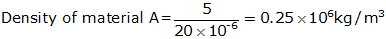For material B:

Mass =20kg

Volume =90 cm3 = 90x 10-6m3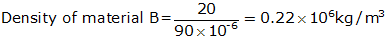Density of material A is more than density of material B.

Question 55

(a) Define buoyant force. Name two factors on which buoyant force depends.

(b) What is the cause of buoyant force?

(c) When a boat is partially immersed in water, it displaces 600kg of water. How much is the buoyant force acting on the boat in newton? (g=10m/s2)

Solution 55

(a) The upward force acting on an object immersed in a liquid is called buoyant force.

Factors affecting buoyant force:

(i) Volume of object immersed in the liquid,

(ii) Density of the liquid.

(b) The cause of buoyant force is the greater upward pressure exerted by water underneath the object..

(c) Mass of water displaced = 600kg

Weight of water displaced, W =m x g

=600 x 10 =6000N

Since, the weight of water displaced by the boat is 6000N, therefore the buoyant force acting on the boat will also be 6000N.

Question 56

(a) State the principle of floatation.

(b) A floating boat displaces water weighing 6000 newton.

(i)What is the buoyant force on the boat?

(ii)What is the weight of the boat?

Solution 56

(a) According to the principle of floatation: An object will float in a liquid if the weight of object is equal to the weight of liquid displaced by it.

Weight of object = Weight of liquid displaced by it.

(b) Weight of water displaced by boat= 6000N

(i) Buoyant force =6000N, as the weight of water displaced is equal to buoyant force.

(ii) Weight of a floating object = Weight of water displaced by it = 6000N

Question 57

(a) Define density. What is the SI unit of density?

(b) Define relative density. What is the SI unit of relative density?

(c) The density of turpentine is 840kg/m3. What will be its relative density? (density of water=1000kg/m3)

Solution 57

(a) The density of a substance is defined as mass of the substance per unit volume.SI unit of density is kg/m3.

(b) Relative density of a substance is the ratio of its density to that of water.Relative density has no units as it is a ratio of two similar quantities.

(c) Density of turpentine =840kg/m3

Density of water=1000kg/m3Question 58

(a) Define pressure.

(b) What is the relation between pressure, force and area?

(c) Calculate the pressure when a force of 200N is exerted on an area of:

(i)10 m2

(ii)5 m2

Solution 58

(a) Pressure is the force acting perpendicularly on a unit area of the object.

(b)(c) (i) Pressure on an area of 10 m2

Force =200N(ii) Pressure on an area of 5 m2

Force =200N## Chapter 3 - Gravitation Exercise 126

Question 59

(a) What are fluids? Name two common fluids.

(b) State Archimedes' Principle.

(c) When does an object float or sink when placed on the surface of liquid?

Solution 59

(a) Those substances which can flow easily are called fluids. All the liquid and gases are fluids, like water, air etc.

(b) Archimedes' Principle :

When an object is wholly (or partially) immersed in a liquid, it experiences a buoyant force (or upthrust) which is equal to the weight of liquid displaced by the object.

Buoyant force on an object = weight of liquid displaced by that object

(c) If the buoyant force exerted by the liquid is less than the weight of the object, the object will sink in the liquid. If the buoyant force exerted by the liquid is equal to or greater than the weight of the object, the object will float in the liquid.

Question 60

(a) How does a boat float in water?

(b) A piece of steel has a volume of 12cm3, and a mass of 96 g. What is its density:

(i) In g/cm3?

(ii) In kg/m3?

Solution 60

(a) A floating boat displaces water equal to its own weight. This displaced water exerts buoyant force to balance the weight of boat and keep it afloat.

(b) (i) Mass = 96 g

Volume = 12cm3(ii) Mass = 96 x 10-3kg

Volume =12 x 10-6m3Question 61

An elephant weighing 40,000N stands on one foot of area 100cm2 whereas a girl weighing 400N is standing on one 'stiletto' heel of area 1 cm2.

(a) Which of the two, elephant or girl, exerts a larger force on the ground and by how much?

(b) What pressure is exerted on the ground by the elephant standing on one foot?

(c) What pressure is exerted on the ground by the girl standing on one heel?

(d) Which of the two exerts larger pressure on the ground: elephant or girl?

(e) What is the ratio of pressure exerted by the girl to the pressure exerted by the elephant?

Solution 61

Weight of elephant=40000N

Area of one foot =1000 cm2= 1000 x 10-4m2

Weight of girl=400N

Area of heel of girl =1 cm2=1 x 10-4m2

(a) Elephant has a larger weight of 40000N, therefore, elephant exerts a larger force on the ground. Elephant exerts a larger force on the ground by 40000N - 400 N=39600N.

(b) Weight of elephant = 40000N

Area of one foot =1000cm2= 1000 x 10-4m2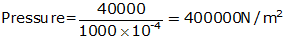(c) Weight of the girl = 400N

Area of heel of girl = 1 cm2 = 1 x 10-4m2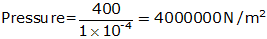(d) Girl exerts a larger pressure on the ground.

(e)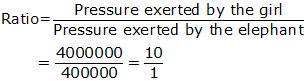The pressure exerted by girl is 10 times greater than that exerted by the elephant.

## Chapter 3 - Gravitation Exercise 127

Question 72

If two equal weights of unequal volumes are balanced in air, what will happen when they are completely dipped in water? Why?

Solution 72

The two equal weights of unequal volumes which are balanced in air, will get imbalanced when they are completely dipped in water because due to their unequal volumes, they will displace unequal volumes of water and hence suffer unequal loss in weight.

Question 73

Two different bodies are completely immersed in water and undergo the same loss in weight. Is it necessary that their weights in air should also be the same? Explain.

Solution 73

No, it is not necessary that their weights in air should also be the same. This is because the two bodies have undergone the same loss in weight on completely immersing in water due to their equal volumes and not because of their equal weights, so they may have different weights in air.

Question 74

A body floats in kerosene of density 0.8 x 103kg/m3 up to a certain mark. If the same body is placed in water of density 1.0 x 103 kg/m3, will it sink more or less? Give reason for your answer.

Solution 74

The body will sink less in water. This is because the density of water is more than that of kerosene due to which water will exert a greater upward buoyant force on the body.

Question 75

Giving reasons state the reading on a spring balance when it is attached to a floating block of wood which weighs 50 g in air.

Solution 75

The reading on spring balance will be zero. This is because the weight of floating block of wood is fully supported by the liquid in which it is floating and hence it does not exert any force on the spring balance.

Question 76

If a fresh egg is put into a beaker filled with water, it sinks. On dissolving a lot of salt in the water, the egg begins to rise and then floats. Why?

Solution 76

When a lot of salt is dissolved in water, then the density of salt solution becomes much more than pure water. Due to its much higher density, the salt solution exerts a greater upward buoyant force on the egg making it rise and then float.

## Chapter 3 - Gravitation Exercise 128

Question 77

A beaker full of water is suspended from spring balance. Will the reading of the balance change:

(a) If a cork is placed in water?

(b) If a piece of heavy metal is placed in it?

Solution 77

(a) The reading of spring balance will not change if a cork is placed in water because cork, being lighter than water, floats in water.

(b) The reading of spring balance will change if a piece of heavy metal is placed in water because heavy metal being denser than water, sinks in water.

Question 78

When a golf ball is lowered into a measuring cylinder containing water, the water level rises by 30cm3 when the ball is completely submerged. If the mass of ball in air is 33g, find its density.

Solution 78

Volume of golf ball = rise in water level = 30 cm3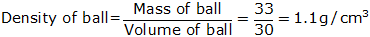Question 79

A boy gets into a floating boat.

(a) What happens to the boat?

(b) What happens to the weight of water displaced?

(c) What happens to the buoyant force on the boat?

Solution 79

(a) The boat sinks a little more in water, that is, the boat floats lower in water.

(b) The weight of water displaced (by the submerged part of the boat) increases.

(c) The buoyant force acting on the boat increases.

Question 80

A half kg sheet of tin sinks in water but if the same sheet is converted into a box or boat, it floats. Why?

Solution 80

The sheet of tin sinks in water because the density of tin is higher than that of water. When the same sheet of tin is converted into a box or a boat, then due to the trapping of lot of 'light' air in the box or boat, the average density of the box or boat made of tin sheet becomes lower than that of water and hence it floats in water.